# Constructing a Correlation matrix

The topic of this issue was inspired by a support inquiry: how can I construct a correlation matrix for my N assets returns efficiently?

NumXL comes with numerous functions to compute the cross-correlation between two time-series, but how can we do so for, say, 20 variables without any intermediate calculations? Not to worry, Excel has a few functions: MATCH(.) and INDEX(.), that we can use in our formulas to make constructing a correlation matrix easy and quick. This is exactly what we will cover in this issue.

In sum, we wish to transform the following input data table: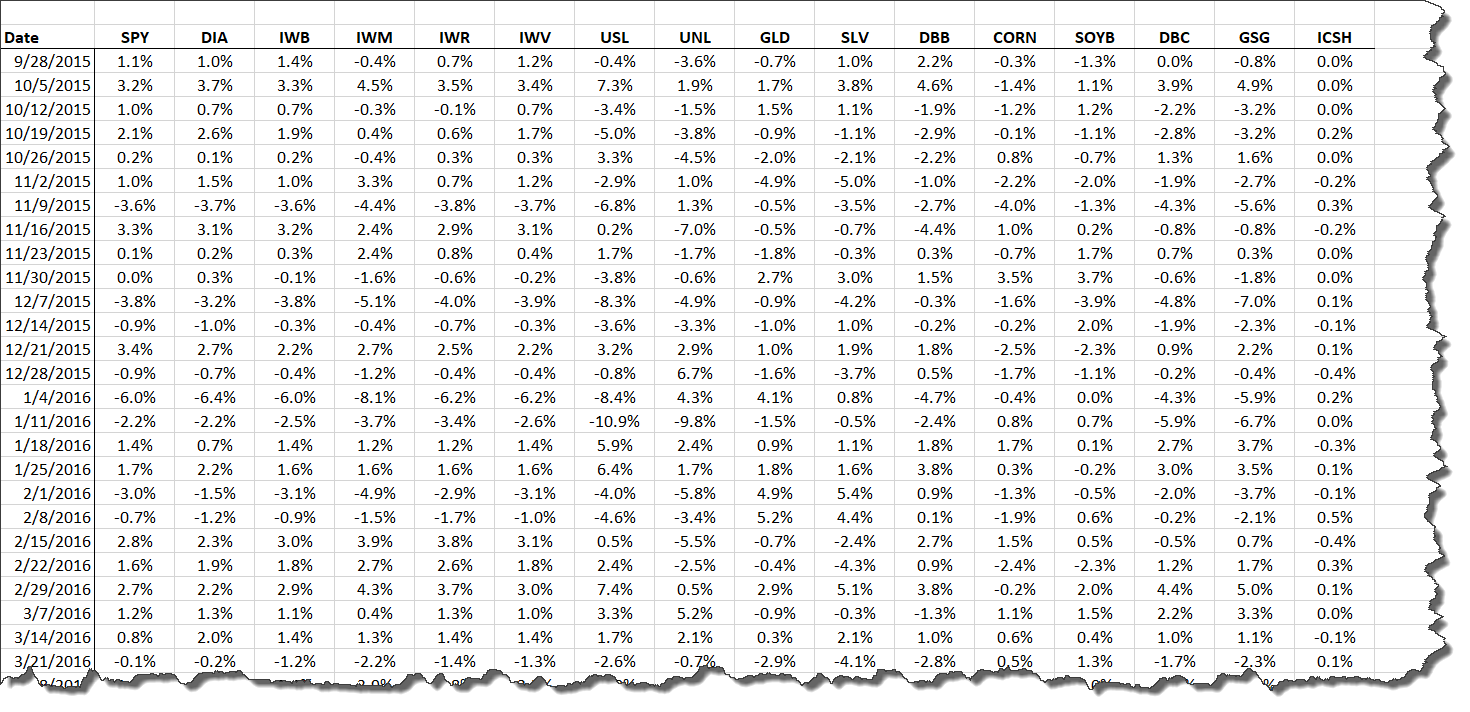Into this correlation matrix: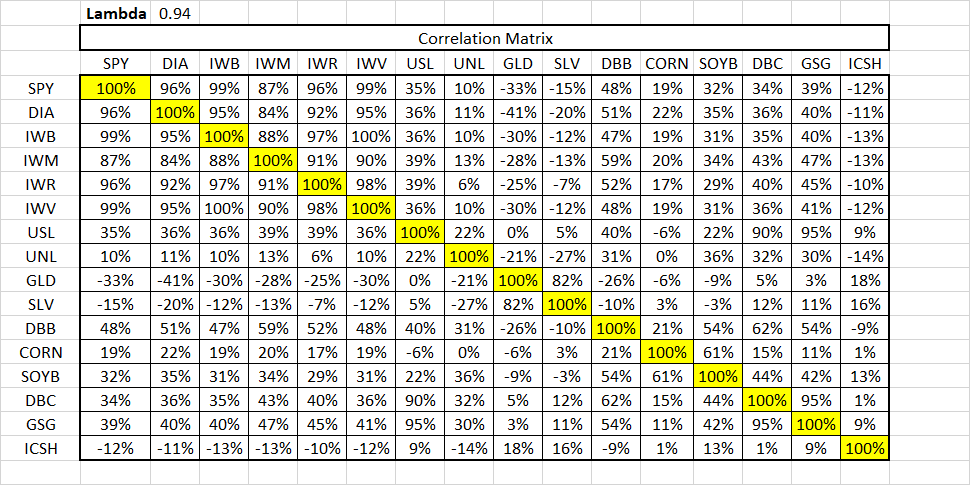Interested? Let’s get to it!

## Data Preparation

In this tutorial, we will use the logarithmic weekly returns of 16 ETFs for the period between September 28, 2015, and February 17th, 2020 (just before the COVID-19 epidemic market volatility). We chose the 16 ETFs to cover the US equity market (e.g., S&P 500, Dow Jones Industrial Average, Russell 1000/2000/3000, etc.), US Energy market (Oil and Natural Gas), Metals ( Gold, Silver, and industrial base metals), and finally, ultra-short debt market (cash).

Next, we copied the log weekly returns in a separate worksheet, each in a separate column, but aligned all assets using a common date field (column A).

Now, let’s define a name for the cells-range of the data table:

• Select or switch to the Formulas tab
• Select the input cell range, and then click on “Define Name”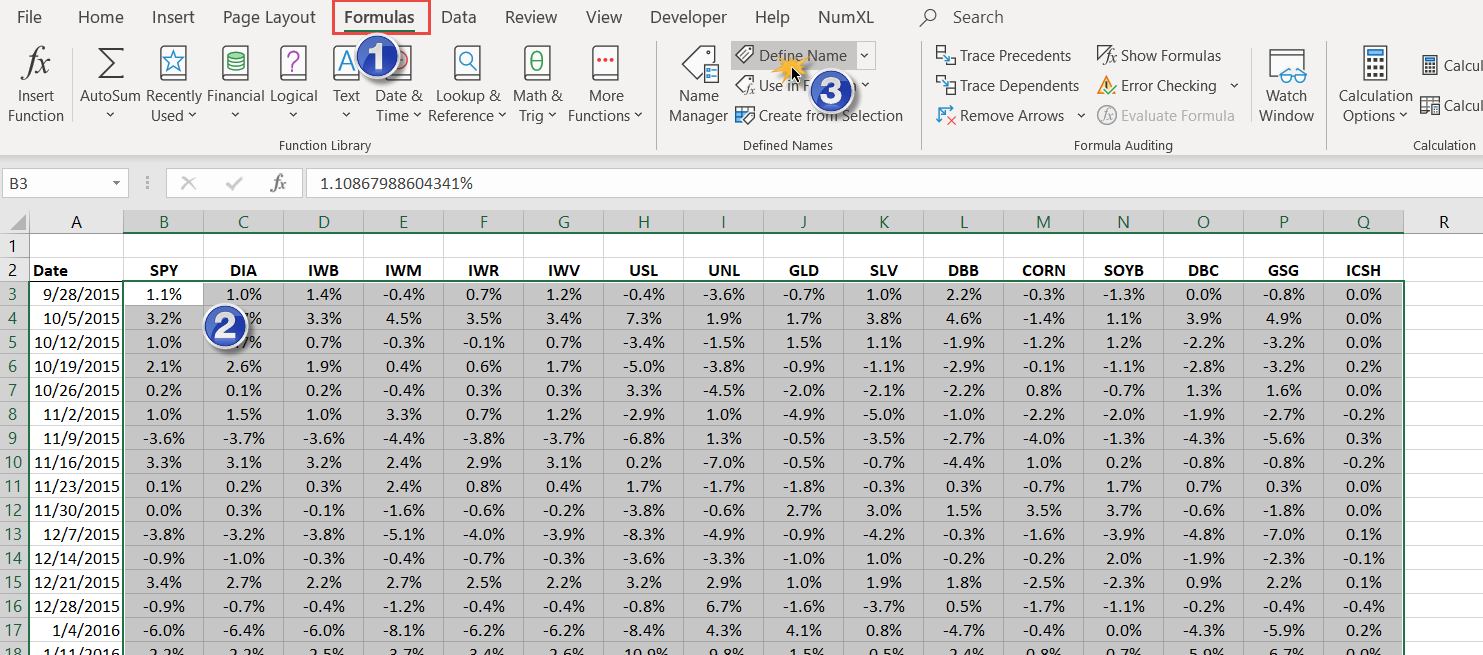• The “Define Name” dialog pops up, with a few fields pre-populated, e.g., “Refer to” has the data table cells range, and the scope is set to “Workbook”).
• Change the Name in the “Define Name” dialog to something meaningful, so we chose “RETURNS”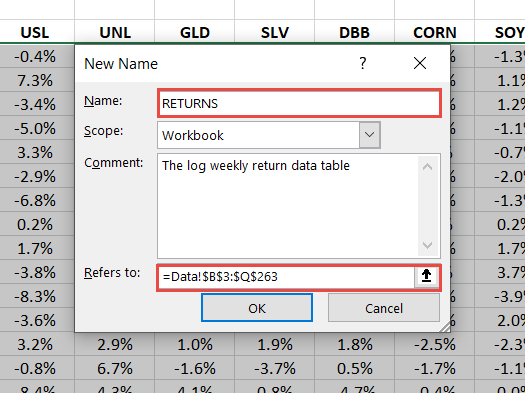• Click OK.

Let’s create another defined name (say, SYMBOLS”) for assets symbol tickers (row 2). Repeat the same steps as above but for the top row with ticker symbols.

Now, if you examine the Name Manager, you should have the following: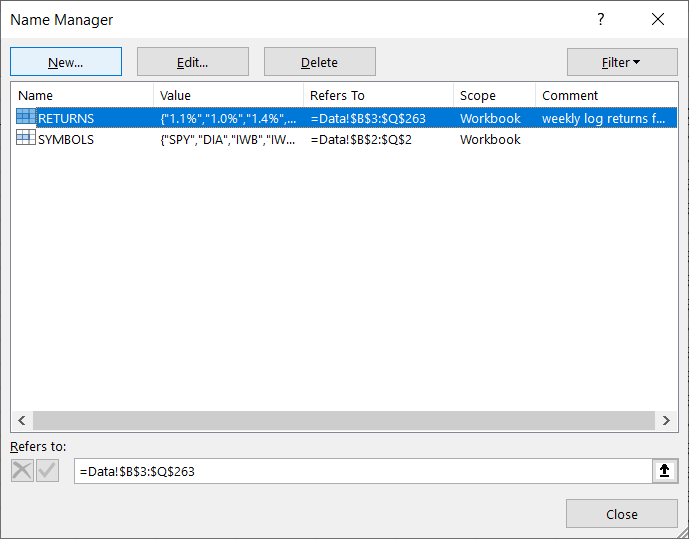## Correlation Matrix

For a correlation matrix, each row or each column corresponds to a single asset, so we will set the ticker symbols as the column and row headers of our correlation matrix.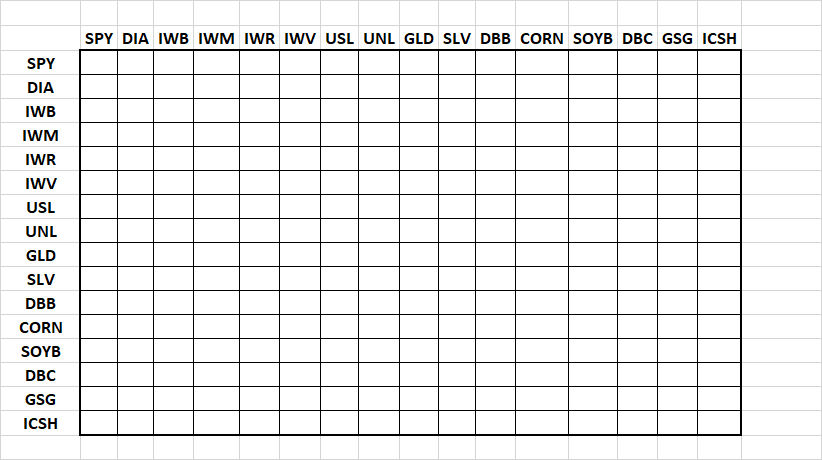Hint: You can use the “transpose” feature in “Paste options”, to paste a copied row (e.g., ticker symbols row) into a column.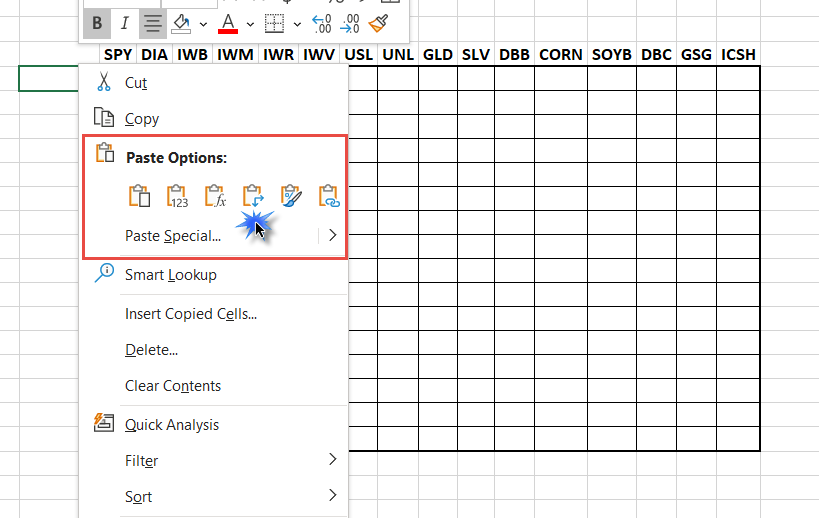## Time-Series Referencing

In the correlation table, each cell represents the cross-correlation between the two assets returns: column and row. For instance, in the figure below, the grey-cell is the correlation between IWB and DIA.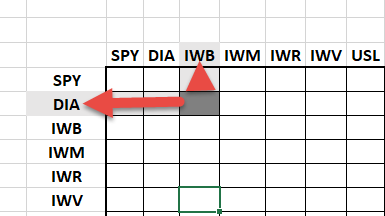Now, we need to use the ticker symbol to reference the time series in the data table:

Step 1: Covert the ticker symbol into a numeric offset. Use the MATCH(.) Function to evaluate the index of the ticker in the SYMBOLS defined name. We do that for both the column and row header. For Example:

MATCH("DIA", SYMBOLS, 0) = 2

MATCH(“IWB”, SYMBOLS, 0) = 3

Step 2: Reference the corresponding time series

Use the INDEX(.) function to reference one column, but all rows, in the RETURNS defined name. We do that for both the column and row header but using its offset.

INDEX ( RETURNS, , 3) = IWB time series

INDEX ( RETURNS, , 2 ) = DIA time series

Step 3: Combine the MATCH(.) and the INDEX(.) functions

INDEX (RETURNS , ,MATCH (“IWB”, SYMBOLS, 0)) = IWB time series

INDEX (RETURNS , ,MATCH (“DIA”, SYMBOLS, 0)) = DIA time series

Step 4: Choose your favorite correlation function, pass the two time-series, and store the returned value in the table.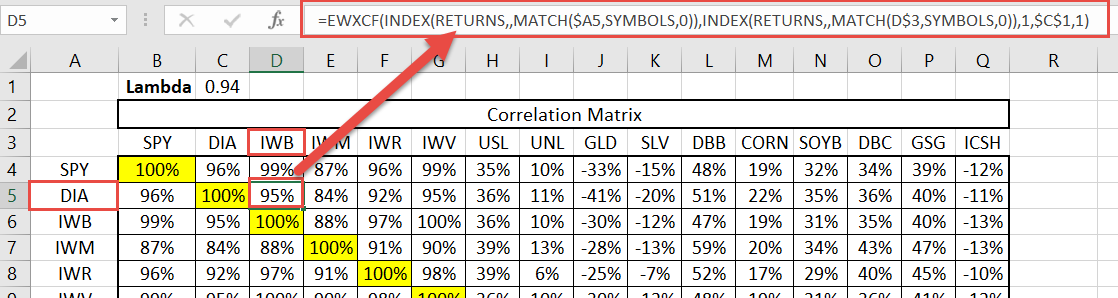Step 5: Copy the formula above to other cells (rows and columns) to calculate the whole correlation matrix.## Conclusion

In this tutorial, we used the built-in excel functions: MATCH(.) and INDEX(.), and constructed a correlation matrix without any intermediate calculation, in just a few simple steps. Additionally, we used the “defined names” feature to encapsulate the data set, and simplified not only the formulas but the maintenance of the input data as well: adding observations and/or assets.